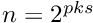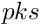GetFEM  5.4.2
dal::dynamic_array< T, pks > Class Template Reference

Dynamic Array. More...

`#include <dal_basic.h>`

## Public Member Functions

size_type size (void) const
Number of allocated elements.

bool empty (void) const
True if no space is allocated.

iterator begin (void)
Iterator on the first element.

const_iterator begin (void) const
Constant iterator on the first element.

iterator end (void)
Iterator on the last + 1 element.

const_iterator end (void) const
Constant iterator on the last + 1 element.

void clear (void)
Clear and desallocate all the elements.

const_reference operator[] (size_type ii) const
Gives a constant reference on element ii.

reference operator[] (size_type ii)
Gives a reference on element ii.

size_type memsize (void) const
Gives the total memory occupied by the array.

void swap (size_type i1, size_type i2)
Swap element i1 and i2.

## Detailed Description

### template<class T, unsigned char pks> class dal::dynamic_array< T, pks >

Dynamic Array.

Defines the basic container of the library which is dal::dynamic_array<T, pks>. This container is virtually an infinite array of element of type T. When a random acces tab[i] is called, a control is made on i and an allocation is made if needed. The allocation is made by blocks of n elements, where.is an optional parameter assumed to be 5. The structure of this container is similar to the one of std::deque<T> but with a faster random access.

### Example of code

If T is any type (with or without trivial constructor/destructor, and with constructor T(0) and T(1)), the following code is valid:

#include<dal_basic.h>
tab = T(0); // 51 elements in tab.
std::fill(tab.begin(), tab.end(), T(0)); // 51 elements initialized
for( ; it != ite; ++it)
{ *it = T(1); } // only the 50 first elements are changed.

Definition at line 47 of file dal_basic.h.

The documentation for this class was generated from the following file:
dal_basic.h
Dynamic array class.
dal::dynamic_array::begin
iterator begin(void)
Iterator on the first element.
Definition: dal_basic.h:225
dal::dynamic_array
Dynamic Array.
Definition: dal_basic.h:47
dal::dna_iterator
Iterator class for dynamic array.
Definition: dal_basic.h:50
dal::dynamic_array::end
iterator end(void)
Iterator on the last + 1 element.
Definition: dal_basic.h:229